1800-1023-196

+91-120-4616500

# Limitations of pH scale

## Limitations of pH Scale

(i) pH values of the solutions do not give us immediate idea of the relative strengths of the solutions. A solution of pH =1 has a hydrogen ion concentration 100 times that of a solution pH = 3 (not three times). A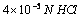is twice concentrated of a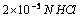solution, but the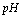values of these solutions are 4.40 and 4.70 (not double).

(ii) pH value zero is obtained in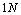solution of strong acid. If the concentration is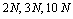, etc. the respective pH values will be negative.

(iii) A solution of an acid having very low concentration, say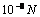, can not have pH 8, as shown by pH formula but the actual pH value will be less than 7.

pK value: p stands for negative logarithm. Just as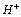and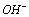ion concentrations range over many negative powers of 10, it is convenient to express them as pH or pOH, the dissociation constant (K) values also range over many negative powers of 10 and it is convenient to write them as pK. Thus, pK is the negative logarithm of dissociation constant.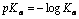and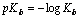Weak acids have higher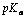values. Similarly weak bases have higher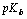values

For any conjugate acid-base pair in aqueous solution,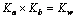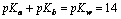(at 298o K)

Calculation of the pH of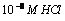&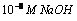If we use the relation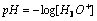we getequal to 8, but this is not correct because an acidic solution connot havegreater than 7. In this conditionconcentration of water cannot be neglected.

Therefore,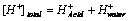Since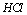is strong acid and completely ionised,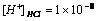,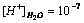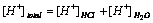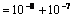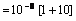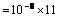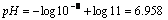Similarly if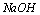concentration is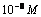Then,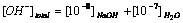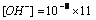;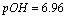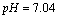## NEET & AIIMS Exam Sample Papers

 AIIMS SAMPLE PAPERS View More NEET SAMPLE PAPERS View More